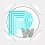## Q. Using the myarray4 created in the above questions, write commands for the following:

a) Find the sum of all elements.

b) Find the sum of all elements row wise.

c) Find the sum of all elements column wise.

d) Find the max of all elements.

e) Find the min of all elements in each row.

f) Find the mean of all elements in each row.

g) Find the standard deviation column wise.

(a)

To find the sum of all elements in the array myarray4, you can use the np.sum() function in NumPy.

```myarray4 = np.arange(-1, -1 + 14 * 3 * 0.25, 0.25).reshape(14, 3)

#sum of all elements in myarray4
sum_of_elements = np.sum(myarray4)

print("Sum of all elements:", sum_of_elements)
```

(b)

To find the sum of elements row-wise in the array myarray4, you can use the np.sum() function with the axis parameter set to 1. This will calculate the sum along each row.

```myarray4 = np.arange(-1, -1 + 14 * 3 * 0.25, 0.25).reshape(14, 3)

#sum of elements row-wise in myarray4
row_sums = np.sum(myarray4, axis=1)

print("Sum of elements row-wise:")
print(row_sums)
```

(c)

To find the sum of elements column-wise in the array myarray4, you can use the np.sum() function with the axis parameter set to 0. This will calculate the sum along each column.

```myarray4 = np.arange(-1, -1 + 14 * 3 * 0.25, 0.25).reshape(14, 3)

#sum of elements column-wise in myarray4
column_sums = np.sum(myarray4, axis=0)

print("Sum of elements column-wise:")
print(column_sums)
```

(d)

To find the maximum value among all elements in the array myarray4, you can use the np.max() function in NumPy.

```myarray4 = np.arange(-1, -1 + 14 * 3 * 0.25, 0.25).reshape(14, 3)

# the maximum value among all elements in myarray4
max_value = np.max(myarray4)

print("Maximum value:", max_value)
```

(e)

To find the minimum value among all elements in each row of the array myarray4, you can use the np.min() function with the axis parameter set to 1. This will calculate the minimum value along each row.

```myarray4 = np.arange(-1, -1 + 14 * 3 * 0.25, 0.25).reshape(14, 3)

# the minimum value among all elements in each row of myarray4
row_mins = np.min(myarray4, axis=1)

print("Minimum value in each row:")
print(row_mins)
```

(f)

```myarray4 = np.arange(-1, -1 + 14 * 3 * 0.25, 0.25).reshape(14, 3)

# the Mean  value in each row of myarray4
row_mean = np.mean(myarray4, axis=1)

print("mean value in each row:")
print(row_mean)
```

(g)

To find the standard deviation column-wise in the array myarray4, you can use the np.std() function with the axis parameter set to 0. This will calculate the standard deviation along each column.

```myarray4 = np.arange(-1, -1 + 14 * 3 * 0.25, 0.25).reshape(14, 3)

# the standard deviation column-wise in myarray4
column_stdevs = np.std(myarray4, axis=0)

print("Standard deviation column-wise:")
print(column_stdevs)
```

You can help us by Clicking on ads. ^_^
Please do not send spam comment : )

1.yoo moderator bro or sis, we want to be friends with you, please reply

1.